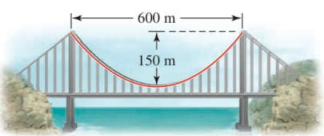Chapter 12.1, Problem 63E### Algebra and Trigonometry (MindTap ...

4th Edition
James Stewart + 2 others
ISBN: 9781305071742

#### Solutions

Chapter
Section### Algebra and Trigonometry (MindTap ...

4th Edition
James Stewart + 2 others
ISBN: 9781305071742
Textbook Problem

# APPLICATIONS Suspension Bridge In a suspension bridge the shape of the suspension cables is parabolic. The bridge shown in the figure has towers that are 600 m apart, and the lowest point of the suspension cables is 150 m below the top of the towers. Find the equation of the parabolic part of the cables, placing the origin of the coordinate system at the vertex. [Note: This equation is used to find the length of cable needed in the construction of the bridge.]To determine

The equation of parabolic section of the bridge is shown in figure (1).

Explanation

Given:

A suspension bridge with its cable is parabolic shape is as shown in figure (1).

Figure (1)

Distance between the two towers of the bridge is 600m and the lowest point of the cables from the top of towers is 150m.

Concept:

The general equation of a parabola with vertical axis where vertex V(0,0) and focus at F(0,p) is given by,

x2=4py …… (1)

Where y=p is the directrix.

1) If p<0, then parabola opens downward.

2)If p>0, then parabola opens upward.

Width of a parabola is given by 2|x|.

Height of a parabola is given by 2|y|.

Calculation:

Since, given parabola is drawn with vertical axis, the general equation will be given as,

x2=4py

As the parabola opens upward, p>0

### Still sussing out bartleby?

Check out a sample textbook solution.

See a sample solution

#### The Solution to Your Study Problems

Bartleby provides explanations to thousands of textbook problems written by our experts, many with advanced degrees!

Get Started